ExamplesNext: Freeing Requests Up: Nonblocking Communication Previous: Completion Operations

Examples

We illustrate the use of nonblocking communication for the same Jacobi computation used in previous examples (Example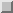-). To achieve maximum overlap between computation and communication, communications should be started as soon as overlap possible and completed as late as possible. That is, sends should be posted as soon as the data to be sent is available; receives should be posted as soon as the receive buffer can be reused; sends should be completed just before the send buffer is to be reused; and receives should be completed just before the data in the receive buffer is to be used. Sometimes, the overlap can be increased by reordering computations. Jacobi, using nonblocking

```Example2.16 Using nonblocking communications in Jacobi computation.
...
REAL, ALLOCATABLE A(:,:), B(:,:)
INTEGER req(4)
INTEGER status(MPI_STATUS_SIZE, 4)
...
! Compute number of processes and myrank
CALL MPI_COMM_SIZE(comm, p, ierr)
CALL MPI_COMM_RANK(comm, myrank, ierr)

! compute size of local block
m = n/p
IF (myrank.LT.(n-p*m)) THEN
m = m+1
END IF

! Compute neighbors
IF (myrank.EQ.0) THEN
left = MPI_PROC_NULL
ELSE
left = myrank - 1
END IF
IF (myrank.EQ.p-1)THEN
right = MPI_PROC_NULL
ELSE
right = myrank+1
END IF

! Allocate local arrays
ALLOCATE (A(0:n+1,0:m+1), B(n,m))
...
!Main Loop
DO WHILE(.NOT.converged)
! compute
DO i=1, n
B(i,1)=0.25*(A(i-1,j)+A(i+1,j)+A(i,0)+A(i,2))
B(i,m)=0.25*(A(i-1,m)+A(i+1,m)+A(i,m-1)+A(i,m+1))
END DO

! Communicate
CALL MPI_ISEND(B(1,1),n, MPI_REAL, left, tag, comm, req(1), ierr)
CALL MPI_ISEND(B(1,m),n, MPI_REAL, right, tag, comm, req(2), ierr)
CALL MPI_IRECV(A(1,0),n, MPI_REAL, left, tag, comm, req(3), ierr)
CALL MPI_IRECV(A(1,m+1),n, MPI_REAL, right, tag, comm, req(4), ierr)

! Compute interior
DO j=2, m-1
DO i=1, n
B(i,j)=0.25*(A(i-1,j)+A(i+1,j)+A(i,j-1)+A(i,j+1))
END DO
END DO
DO j=1, m
DO i=1, n
A(i,j) = B(i,j)
END DO
END DO

! Complete communication
DO i=1, 4
CALL MPI_WAIT(req(i), status(1.i), ierr)
END DO
...
END DO
```
The next example shows a multiple-producer, single-consumer code. The last process in the group consumes messages sent by the other processes. producer-consumer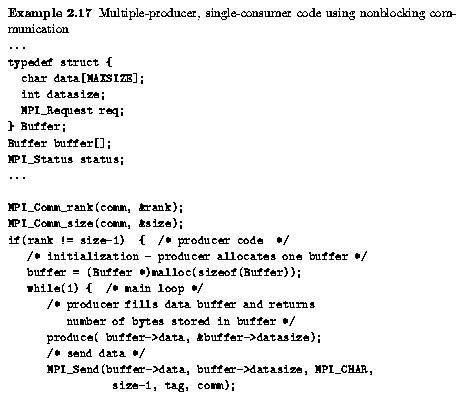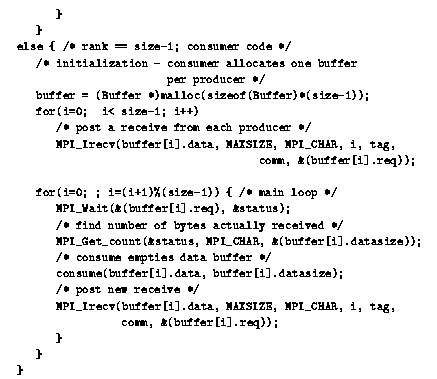The example imposes a strict round-robin discipline, since round-robin the consumer receives one message from each producer, in turn. In some cases it is preferable to use a ``first-come-first-served'' discipline. This is achieved by using MPI_TEST, rather than MPI_WAIT, as shown below. Note that MPI can only offer an first-come-first-served approximation to first-come-first-served, since messages do not necessarily arrive in the order they were sent.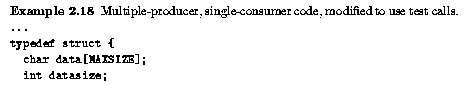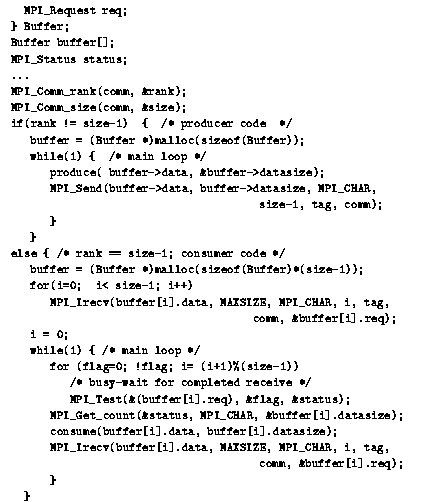Jack Dongarra
Fri Sep 1 06:16:55 EDT 1995